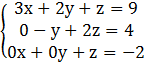### Sample Problem

Solve using back substitution:x=

y=

z=

#### Solution

Using the 3rd equation, where z is given, to back substitute into the 2nd equation (plug in z=-2)

-y+2z=4

-y+2(-2)=4

-y-4=4

-y=8 ,  y=-8

Then substitute y=-8 and z=-2 into Equation 1:

3x+2(-8)+(-2)=9

3x-16-2=9

3x=27

x=9

x=9 ; y=-8 ; z=-2